Open in App
Not now

# Underscore.js _.map() Function

• Last Updated : 26 Oct, 2022

The Underscore.js is a JavaScript library that provides a lot of useful functions that helps in the programming in a big way like the map, filter, invoke etc even without using any built-in objects. The _.map() function is an inbuilt function in Underscore.js library of the JavaScript which is used to produce a new array of values by mapping each value in list through transformation function (iterate). It displays the result as a list on the console. In this list the output of the first element, i.e, the element at index 0 has it’s result at index 0 of the returned list and when all the elements of the list are passed to the function/iterate and no more elements remain then the _.map loop ends. Syntax:

`_.map(list, function)`

Parameters: It accepts two parameters which are specified below-

• list: It is the list which contains some elements.
• function: It is the function which is executed by taking each element of the list.

Return values: It returns the object property value or array element value at the current position.

• Passing a list of numbers with user defined function: When we are passing the list of elements to the user defined function then it takes the element from the list one by one and performs the operations of the function. Here, the function calculates the multiplication operation. It multiplies the list elements by 2. After performing its operations, the function then returns the value to be shown on the console.

## html

 `<``html``>` ` `  `<``head``>` `    ``<``script``>` `        ``<``src` `= "https://cdnjs.cloudflare.com/ajax/libs/` `                ``underscore.js/1.9.1/underscore-min.js" >` `    ``` `    ``<``script` `type="text/javascript"` `    ``src="https://cdnjs.cloudflare.com/ajax/libs/underscore.js` `         ``/1.9.1/underscore-min.js.map">` ` `  `    ``<``script` `type="text/javascript"` `     ``src="https://cdnjs.cloudflare.com/ajax/libs/underscore.js` `          ``/1.9.1/underscore.js">` `` ` `  `<``body``>` `    ``<``script` `type="text/javascript">` `         ``_.map([1, 2, 3, 4], function(num){ return num * 2; });` `    ``` `` ` `  ``

• Output: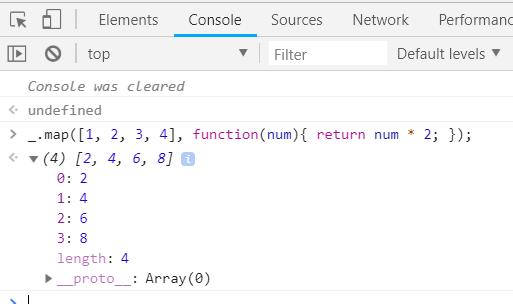• Passing a list of numbers with another user defined function: When we are passing the list of numbers to the user defined function which takes ‘num’ as argument then it takes the numbers from the list one by one and performs it’s operation. The function used here takes each element from the list and uses it in the resulted sentence. Therefore, the final result is a sentence “This is **(element of the list) list item”.

## html

 `<``html``>` ` `  `<``head``>` `    ``<``script``>` `        ``<``src` `= "https://cdnjs.cloudflare.com/ajax/libs/` `                ``underscore.js/1.9.1/underscore-min.js" >` `    ``` `    ``<``script` `type="text/javascript"` `    ``src="https://cdnjs.cloudflare.com/ajax/libs/underscore.js` `         ``/1.9.1/underscore-min.js.map">` ` `  `    ``<``script` `type="text/javascript"` `     ``src="https://cdnjs.cloudflare.com/ajax/libs/underscore.js` `          ``/1.9.1/underscore.js">` `` ` `  `<``body``>` `    ``<``script` `type="text/javascript">` `         ``_.map( [1, 2, 3], function( num ) {` `                 ``for(var i=0;i<``num``;i++) ` `                      ``var str="This is";` `                      ``str+=i+1;` `                      ``str+="list item";` `                      ``return(str);` `         ``});` `    ``` `` ` `  ``

• Output: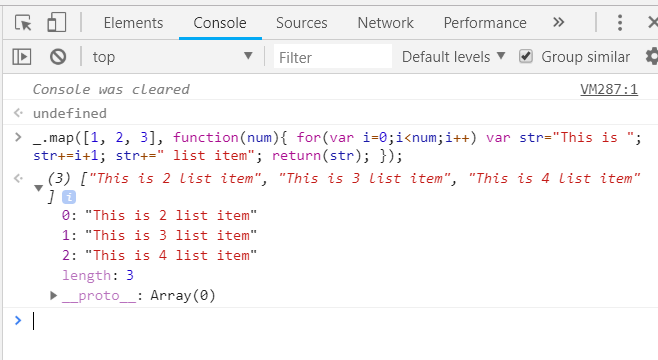• Passing a list of numbers with _.last inbuilt function: When we are passing the list of numbers to the _.last inbuilt function then it takes the words from the list one by one and performs it’s operation. The _.last() takes each array from the list and returns the last element from each array. Therefore, the final result is the last element of every array.

## html

 `<``html``>` ` `  `<``head``>` `    ``<``script``>` `        ``<``src` `= "https://cdnjs.cloudflare.com/ajax/libs/` `                ``underscore.js/1.9.1/underscore-min.js" >` `    ``` `    ``<``script` `type="text/javascript"` `    ``src="https://cdnjs.cloudflare.com/ajax/libs/underscore.js` `         ``/1.9.1/underscore-min.js.map">` ` `  `    ``<``script` `type="text/javascript"` `     ``src="https://cdnjs.cloudflare.com/ajax/libs/underscore.js` `          ``/1.9.1/underscore.js">` `` ` `  `<``body``>` `    ``<``script` `type="text/javascript">` `         ``_.map([[1, 2], [3, 4], [5, 6]], _.last);` `    ``` `` ` `  ``

• Output: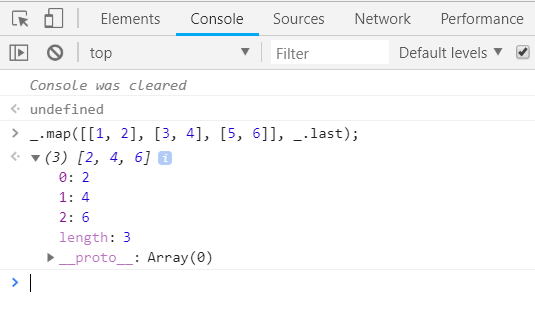• Passing a list of words with a user defined function: First we need to create a list which we will be going to use. Here the function takes each word from the list and prints it to the console along with a set of words which are “is mapped from a list”. The output will contain the list item along with the given set of words.

## html

 `<``html``>` ` `  `<``head``>` `    ``<``script``>` `        ``<``src` `= "https://cdnjs.cloudflare.com/ajax/libs/` `                ``underscore.js/1.9.1/underscore-min.js" >` `    ``` `    ``<``script` `type="text/javascript"` `    ``src="https://cdnjs.cloudflare.com/ajax/libs/underscore.js` `         ``/1.9.1/underscore-min.js.map">` ` `  `    ``<``script` `type="text/javascript"` `     ``src="https://cdnjs.cloudflare.com/ajax/libs/underscore.js` `          ``/1.9.1/underscore.js">` `` ` `  `<``body``>` `    ``<``script` `type="text/javascript">` `         ``var list = ['Geeks','for', 'Geeks', 'JS'];` `         ``m = _.map(list, function (l) {` `               ``return l + ' is mapped from a list.';` `         ``});` `    ``` `` ` `  ``

• Output: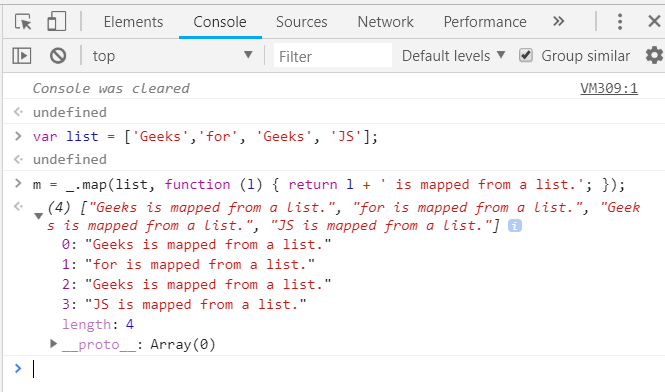• Passing a list of numbers with a : ? function: Pass the list items to the function directly. Here the function uses : ? operators (instead of for loop) to find whether the list number is smaller than or greater than 3 accordingly it prints the result.

## html

 `<``html``>` ` `  `<``head``>` `    ``<``script``>` `        ``<``src` `= "https://cdnjs.cloudflare.com/ajax/libs/` `                ``underscore.js/1.9.1/underscore-min.js" >` `    ``` `    ``<``script` `type="text/javascript"` `    ``src="https://cdnjs.cloudflare.com/ajax/libs/underscore.js` `         ``/1.9.1/underscore-min.js.map">` ` `  `    ``<``script` `type="text/javascript"` `     ``src="https://cdnjs.cloudflare.com/ajax/libs/underscore.js` `          ``/1.9.1/underscore.js">` `` ` `  `<``body``>` `    ``<``script` `type="text/javascript">` `         ``_.map( [ 0, 7, 2, -1, 8 ], function( n ) {` `               ``return n>=3 ? "greater" : "smaller";` `         ``});  ` `    ``` `` ` `  ``

• Output: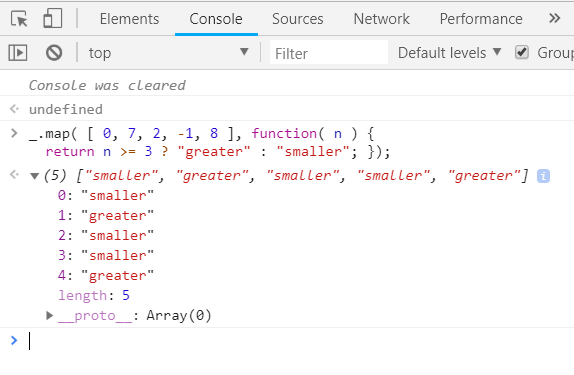My Personal Notes arrow_drop_up
Related Articles